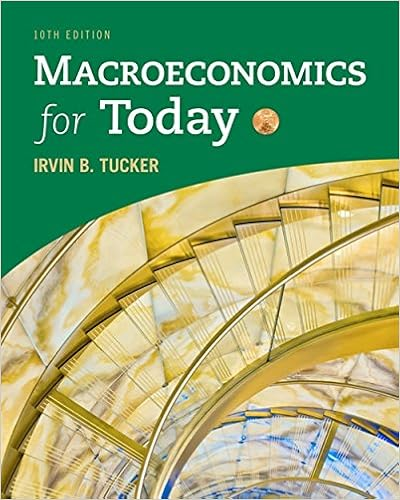# The keynesian cross shows a determination of

• Homework Help
• 6
• 100% (7) 7 out of 7 people found this document helpful

This preview shows page 2 - 4 out of 6 pages.

##### We have textbook solutions for you!
The document you are viewing contains questions related to this textbook.The document you are viewing contains questions related to this textbook.
Chapter 10A / Exercise 12
Macroeconomics for Today
TuckerExpert Verified
8.The Keynesian cross shows: A.determination of equilibrium income and the interest rate in the short run. B.determination of equilibrium income and the interest rate in the long run. C.equality of planned expenditure and income in the short run. D.equality of planned expenditure and income in the long run. (C)
9.The government-purchases multiplier indicates how much ______ change(s) in response to a \$1 change in government purchases.
10.In the Keynesian-cross model, if the MPCequals 0.75, then a \$1 billion increase in government spending increases planned expenditures by ______ and increases the equilibrium level of income by ______.
11.According to the Keynesian-cross analysis, when there is a shift upward in the government-purchases schedule by an amount Gand the planned expenditure schedule by an equal amount, then equilibrium income rises by:
##### We have textbook solutions for you!
The document you are viewing contains questions related to this textbook.The document you are viewing contains questions related to this textbook.
Chapter 10A / Exercise 12
Macroeconomics for Today
TuckerExpert Verified
12.In the Keynesian-cross model, fiscal policy has a multiplied effect on income because fiscal policy: A.increases the amount of money in the economy. B.changes income, which changes consumption, which further changes income. C.is government spending and, therefore, more powerful than private spending. D.changes the interest rate. (B)
13.In the Keynesian-cross model, if taxes are reduced by 100, then planned expenditures ______ for any given level of income.
•••